## conference papersJOURNAL OFAPPLIEDCRYSTALLOGRAPHY
ISSN: 1600-5767
Volume 40| Part s1| April 2007| Pages s73-s77

## A combined small-angle scattering study of a chemical reaction at specific sites and reaction-induced self-assembly as a problem in open non-equilibrium phenomena

aAdvanced Science Research Center, Japan Atomic Energy Agency, Tokai-mura, Ibaraki Pref. 319-1195, Japan, and bDepartment of Polymer Chemistry, Graduate School of Engineering, Kyoto University, Katsura, Kyoto 615-8510, Japan
*Correspondence e-mail: hashimoto.takeji@jaea.go.jp, koizumi.satoshi@jaea.go.jp

(Received 16 August 2006; accepted 22 January 2007; online 23 February 2007)

As a problem in open non-equilibrium phenomena, small-angle scattering (SAS) studies of chemical reactions at specific sites and reaction-induced self-assembly of a system which is obtained by mixing two stable solutions of palladium acetate [Pd(OAc)2] in N,N-dimethylformamide and the second-generation polyamidoamine dendrimer in methanol are presented. The self-assembly was studied using a combination of neutron and X-ray SAS. The results revealed that the self-assembly involves the initial formation of aggregates of an average radius of 20 nm composed of the dendrimers and Pd(OAc)2 followed by formation of palladium nanoparticles of a radius of 2.0 nm inside the aggregates. The aggregates were found to provide a special field for a chemical reaction for reduction of Pd(II) ions with methanol and for the self-assembly of the reduction products of Pd(0) atoms into nanoparticles. The nanoparticles are found to be trapped and stabilized in the aggregates.

### 1. Introduction

Self-assembly of molecules or atoms in open non-equilibrium systems', i.e. systems subjected to external fields, is one of the most important research themes to be addressed in the 21st century from the viewpoints of physical and life sciences. The ordered structures formed in such systems are called dissipative structures', elucidation of which is crucial to understanding pattern formation in nature (Nicolis & Prigogine, 1997; Touring, 1952; Belousov, 1959; Vavilin et al., 1967). Although there have been a number of reports concerning the dissipative structures on macroscopic scales, those on the mesoscopic scales have so far been left unexplored. We would like to emphasize that a combination of small-angle scattering (SAS) methods, which employs ultra-small-angle neutron scattering and X-ray scattering, and small-angle neutron and X-ray scattering (SANS and SAXS) in combination, is quite useful for the studies along this line.

In this paper we would report a combined SANS and SAXS study of the formation of mesoscopic scale dissipative structures in the reaction system shown in Fig. 1. The system comprises two stable solutions as discussed in §2. Mixing the two solutions in the system at a given temperature provides the energy required for a chemical reaction, reduction of palladium ions [Pd(II) ions] with methanol into palladium atoms [Pd(0)] [Pd(OAc)2 + CH3OH → Pd(0) + HCHO + 2CH3COOH] and for self-assembly of the reaction products Pd(0) into Pd nanoparticles. In this sense our system may belong to an open non-equilibrium system.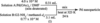Figure 1 Open-nonequilibrium system to be studied in this work which involves chemical reaction and self-assembly upon mixing of two stable solutions A and B.

Since the first successful report by Crooks and his coworkers in 1998 (Zhao et al., 1998), quite a few studies have been reported, focusing on synthesis, structure and also catalytic activity of metal nanoparticles stabilized by dendrimers (Balogh & Tomalia, 1998; Gröhn et al., 2000). Most of the studies in this field deal with the reduction in the solution where dendrimers are molecularly dissolved and metal ions are encapsulated in an interior space of single dendrimers. The reduction of the ions is assumed to give rise to a metal nanoparticle encapsulated and stabilized by the same dendrimer. In this sense, dendrimers provide a special site or field for the chemical reaction and reaction-induced self-assembly. The system to be explored in this work is the same as the systems described above from the point of view that it is composed of dendrimers, metal ions or complex, reducing agent, and solvent, but quite different from the point of view that it forms an association of dendrimers, instead of a stable solution of single isolated dendrimers, possible interpretations of which will be detailed elsewhere (Tanaka et al., 2007). We focus on the roles of dendrimer association on the self-assembly of the metal nanoparticles, which has not been explored so far to the best of our knowledge. We like to note that this work is an extension of the earlier work reported by Naka et al. (2004).

### 2. Experimental methods

#### 2.1. Sample preparation

We first prepared the two stable solutions: the solution A, Pd(OAc)2 in DMF (4.0 × 10−2M) and the solution B, second-generation polyamidoamine dendrimer (PAMAM; G2-NH2, Fig. 2) in deuterated methanol (0.77 × 10−4M). In accordance with Fig. 1, the prescribed amount of the two stable solutions A and B were mixed together and the mixed solution was placed in a bath controlled at 323 K in order to induce a chemical reaction in the system. The concentration ratio r = [Pd(OAc)2]/[-NH2] in the solution was 3.3, where [Pd(OAc)2] and [-NH2] are molar concentrations of Pd(OAc)2 and primary amine groups of the dendrimers in the mixed solution, respectively. The solution, initially transparent yellow, turned to a dark and turbid solution after 24 h, indicating the reduction of Pd(OAc)2 into Pd metal particles.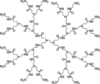Figure 2 Chemical structure of G2.0 PAMAM dendrimer.

#### 2.2. SANS and SAXS measurement

In order to observe the chemical reduction process and the reduction-induced self-assembling process of the system, we conducted time-resolved SANS and SAXS experiments. The SANS experiments were conducted with the SANS-J spectrometer installed at JRR-3 at the Japan Atomic Energy Agency, Tokai, Japan. The average wavelength, λ, of the incident neutron beam was 0.65 nm with distribution of Δλ/λ = 12%. The scattered neutrons were detected by a two-dimensional 3He position-sensitive detector. The obtained scattering patterns were circularly averaged to give scattering intensity profiles as a function of q, where q is the magnitude of the scattering vector, defined by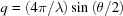with θ being the scattering angle. The obtained scattering intensity profiles were corrected for background scattering, electronic noise of detector, detector sensitivity and transmission, and finally calibrated with a porous aluminium plate to give differential scattering cross-section dΣ(q)/dΩ on the absolute intensity scale (cm−1). The scattering intensity was measured as a function of time t over a time period between tΔt and t after the onset of the chemical reaction where Δt was fixed to be 1 h. The scattering intensity at time t is an average over the period of Δt, which is applied to the SAXS intensity also.

Time-resolved SAXS was measured on a SAXS apparatus which consists of an 18 kW rotating-anode X-ray generator (Bruker AXS K. K., Yokohama, Japan) and a one-dimensional position-sensitive proportional counter. X-rays of a wavelength of λ = 0.154 nm (Cu Kα line monochromated with a graphite crystal) were used. The obtained SAXS profiles were corrected for background scattering and slit-height and slit-width smearings. The absolute SAXS intensity was obtained by the standard nickel foil method (Hendricks, 1972).

### 3. Results

Fig. 3shows the SANS and SAXS profiles (shown by open circles) for the reaction solution at 24 h after onset of the reaction. The figure also contains the weight-averaged SANS and SAXS profiles from the two homogeneous solutions of A and B for references [designated as homogeneous solution1]. In Fig. 3(a), we confirm SANS scattering in excess of that from the homogeneous solutions for the lower q-region of q < 0.3 nm−1. The excess scattering reflects the existence of stable structures larger than 20 nm (≃2π/q, q ≃ 0.3 nm−1) in the reaction medium. In the higher q-region of q > 0.3 nm−1, on the other hand, almost q-independent scattering was observed throughout the reaction process. The observed scattering at the higher q-region (shown by open circles) is equal to the scattering from the homogeneous solutions (shown by + symbols which overlap with open circles) and is considered to be mainly caused by incoherent scattering of the reaction medium as indicated by a dotted straight line. The incoherent scattering unfortunately hides the high-q part of the coherent SANS scattering. However this part of the scattering is fortunately unveiled by SAXS, as shown in Fig. 3(b).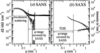Figure 3 (a) SANS and (b) SAXS profiles from the reaction solution obtained at 24 h after mixing before (open circles) and after (filled squares) corrections for the scattering from the homogeneous solution (plus symbols).

The SAXS profiles after the reaction exhibited scattering intensity in excess of that of the homogeneous solutions (shown also by + symbols) in the whole q-range covered by SAXS, indicating that structures of a characteristic length of about 1 nm were built up as will be detailed later. The increase of the scattering intensity from the homogeneous solutions toward higher q is due to thermal diffuse scattering (TDS) arising from acoustic phonons in the system. The TDS intensity level can be estimated from the semi-empirical relationship proposed by Vonk (1973), as shown by a dotted line in Fig. 3(b).

For further analysis of the scattering profiles, we subtracted the incoherent scattering and the TDS from the SANS and SAXS profiles, respectively. In Fig. 3, the corrected SANS and SAXS profiles after subtraction are shown by filled symbols. Fig. 4shows a combined SANS and SAXS profile obtained by a vertical shift of the corrected SANS profile relative to the corrected SAXS profile. The two profiles are smoothly superposed on each other to form a combined SAS profile having the absolute intensity corresponding to the SAXS. The two profiles with overlapping q-regions of 0.15 < q < 0.22 nm−1 make the superposition reliable. The inset shows time changes in the scattering intensity at two representative q values of 0.05 and 0.6 nm−1 indicated by the two arrows on the combined SAS profile, during the reaction process. From the time change in the scattering intensity, we can find that the scattering intensity at q = 0.05 nm−1 is nearly constant with time, while that at q = 0.6 nm−1 increases to more than tenfold with increasing reaction time. The results clearly indicate that the self-assembling process first develops a large structure and then a small structure.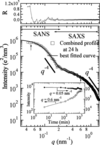Figure 4 Combined SANS and SAXS from the reaction solution obtained at 24 h after mixing (shown by open squares) and the best-fitted theoretical scattering curve (solid line). Inset shows time change in the scattering intensity at q = 0.05 nm−1 and q = 0.6 nm−1 representative of the lower and higher q-regions covered by SANS and SAXS, respectively. R represents the residuals for the best-fitted profile.

### 4. Scattering analysis

We consider a system composed of numbers of nanoparticles entrapped in a template as illustrated in Fig. 5, where the sizes of individual nanoparticle and template are characterized by Rnano and Rtemp, respectively, and the volume fraction of the nanoparticles in the template is given by ϕn. First, we define a shape factor σ(r1) of the template structure such that it is either 1 or 0 when r1 is inside or outside template, respectively. By using the shape factor, the scattering contrast ρ(r1) (scattering length density for SANS or electron density for SAXS) distribution in space is given by ρ(r1) = σ(r1)ρint(r1) where ρint(r1) is the scattering contrast distribution inside the template. According to the scattering theory (Guinier & Fournet, 1955), the scattering field strength, f, from the template is given by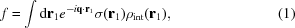where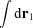indicates the volume integration over all space because of the introduction of σ(r1). Hence, the scattering intensity,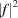can be written by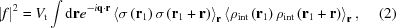where Vt is the volume of the template and r is defined by r = r1r2. We assume here ρint(r) and σ(r) to be independent of each other.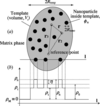Figure 5 Schematic illustration of a scattering model for a template containing Pd nanoparticles (part a) and a spatial distribution of the scattering contrast across l (part b).

The average contrast, ρ0, of a template containing nanoparticles is given by ρ0 = ϕnρn + (1 − ϕn)ρt where ρn and ρt are scattering contrast of nanoparticles and template, respectively [see Fig. 5(b)]. Noting that ρint(r) is given by ρint(r) = ρ0 + η(r) where η(r) indicates scattering contrast fluctuations due to the existence of the nanoparticles inside the template, 〈ρint (r1)ρint(r1 + r)〉r in equation (2)is given by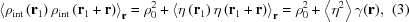where 〈Xr denotes an average of X under a constant r, γ(r) is the correlation function for the scattering contrast fluctuations inside the template, defined by γ(r) ≡ 〈η(r1)η(r1 + r)〉r/〈η2〉, and 〈η2〉 stands for mean-squared contrast fluctuations.

By combining equations (2)and (3), and noting that 〈σ(r1)σ(r1 + r)〉r is equal to the correlation function for the shape of a template Γshape(r),is given by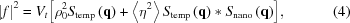where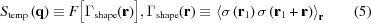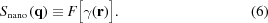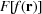indicates the Fourier transform of f(r), and the operator * designates a convolution product. Stemp(q) is the scattering function for a homogeneous template, and Snano(q) reflects scattering arising from a spatial distribution of nanoparticles. When the spatial distribution is liquid-like, Snano(q) can be described by the Percus–Yevick scattering function (Percus & Yevick, 1959), or Snano(q) can be generally given by Debye–Bueche type statistical theory (Debye & Bueche, 1949).

When templates are randomly oriented or both templates and nanoparticles are spherically symmetric, the systems become also spherically symmetric. In this case the scattering intensity I = K|f|2 (where K is a proportionality constant) depends only on |q| = q and is given by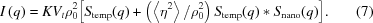Furthermore, when Rtemp >> Rnano, Stemp(q) can be approximated by a delta function δ(q) relative to Snano(q). In this case Stemp(q)*Snano(q) = Snano(q) and hence I(q) is approximated by Iapprox(q),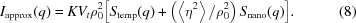Scattering from the complicated structure can be expressed by just a weighted sum of two scattering functions; (i) scattering function for templates and (ii) that for nanoparticles. ρ02 and 〈η2〉 are different for neutron and X-ray. It is important to note that this difference causes a difference in the scattering intensity distribution obtained for neutron and X-ray. The vertical shift of the SANS profile relative to the SAXS profile corresponds to a conversion of ρ02 for SANS into ρ02 for SAXS and thus to a conversion of the SANS profile to the SAXS profile.

Let us consider the simplest case which satisfies the following conditions: (i) both nanoparticles and templates have spherical shape, as inferred from transmission electron microscopy examination (Tanaka et al., 2007); (ii) inter-particle interference effects on Stemp(q) and Snano(q) are insignificant so that they undergo independent scattering, both of which are quite reasonably satisfied from the composition of Pd(OAc)2 and the dendrimer in the reaction solution (Fig. 1). The volume fraction of the dendrimer in the reaction solution is estimated to be quite small, of the order of 10−4. The volume fraction of Pd nanoparticles within the template is also small, smaller than 0.234, judging from [Pd(OAc)2]/[G2-NH2] = 51.9 and the ratio of the volumes of Pd(OAc)2 and [G2-NH2] being of the order of 10−3. Then Stemp(q) and Snano(q) can be rewritten with respect to the form factor of a sphere Φ(qRJ) (J = temp or nano) and Iapprox(q) is given by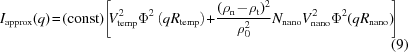where Φ(qR) (R = Rtemp or Rnano) is given by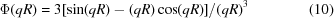and Nnano is numbers of nanoparticles per template. In the case when there is a size distribution, VJ 2 Φ2 (qRJ) (J = temp or nano) should be averaged with respect to normalized distribution functions PJ(R) (J = temp or nano). We assume that PJ(R) is given by the Gaussian distribution with average radius RJ and standard deviation of σJ.

We investigated the validity of the approximated equation [equation (8)] by comparing numerically calculated Iexact(q) and Iapprox(q). Fig. 6presents the results of numerical calculations for Iexact(q) (squares) and Iapprox(q) (solid lines) for five different values of rR = Rtemp/Rnano under the conditions where other parameters are fixed as follows: Rtemp = 20 nm, σnano/Rnano = 0.25, σtemp = 5 nm, 〈η2〉/ρ02 = 0.6. We also fixed the volume fraction of nanoparticles inside the template, ϕn = 0.2 by changing Nnano accordingly. These values represent more or less realistic values in the experimental system. Each profile is vertically shifted to avoid overlapping. It is found that when the ratio, rR, equals 15 and 7.5, Iexact(q) and Iapprox(q) are almost identical. However, in the case of rR = 5.0, Iapprox(q) shows a slightly smaller value than Iexact(q), by a factor 20% in the q-range 0.2 < q < 1.0 nm−1.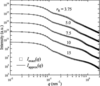Figure 6 Numerically calculated scattering profile for Iexact(q) (open squares) and Iapprox(q) (solid line) for various values of rR, rR = Rtemp/Rnano and for a given set of parameters as described in the text.

### 5. Discussion

#### 5.1. Self-assembling process of the reaction system

In Fig. 4, the solid lines show the predicted curves best-fitted with the experimental profile. It is found that the observed scattering profiles can be well reproduced by the approximated function [equation (8)] for Rtemp = 20 nm and Rnano = 2 nm, satisfying the criterion of rR ≥ 7.5. Thus we can visualize the reaction-induced self-assembling system as follows. Isolated spherical templates are first formed in the solution. Then some Pd(OAc)2, which is relatively weakly associated with the templates, will be chemically reduced slowly to Pd(0) atoms, and the Pd(0) atoms slowly aggregate into Pd nanoparticles inside the templates. It is important to note here that Rtemp is much larger than that of one dendrimer molecule, namely by a factor of 20, because the theoretically calculated radius of gyration of G2-NH2 is ca 1.0 nm (Maiti et al., 2004). This suggests that the template structures are composed of many dendrimers and Pd(OAc)2. This situation is totally different from that encountered in most of the previous relevant studies (Zhao et al., 1998; Balogh & Tomalia, 1998; Gröhn et al., 2000). The time-resolved SAS analysis yielded Rtemp = 20 nm independent of time t but Rnano increased from 0.6 to 2 nm with t. The details will be reported elsewhere (Tanaka et al., 2007).

#### 5.2. Self-assembling mechanism and process of the reaction system

The attractive interactions between Pd(OAc)2 and DMF in solution A and those between the dendrimers and methanol in solution B give a homogeneous solution of A and B, respectively. After mixing the two solutions, Pd(OAc)2 complexes have stronger attractive interactions with dendrimers than a mixed solvent of DMF/methanol of 1/10 v/v. Pd(OAc)2 may associate with various parts of the dendrimers with the association strength increasing in the order of amide groups, tertiary amine and primary amine as presented in Fig. 7(a). Each dendrimer has 16 primary amine, 14 tertiary amine and 28 amide groups so that it can associate with 58 Pd(OAc)2 at maximum. The actual amount of Pd(OAc)2 per G2-NH2 dendrimers in the system prepared according to Fig. 1is 52. Consequently almost all Pd(OAc)2 complexes tend to be associated with and encapsulated within the dendrimers.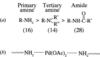Figure 7 Three kinds of nitrogen atoms and order of strength of interactions with Pd(OAc)2. Number in parentheses designate number of each functional group per G2.0 PAMAM dendrimer.

Some of the Pd(OAc)2 complexes which associate with primary amine groups belonging to different dendrimers, as shown in Fig. 7(b), serve as inter-dendrimer binders. This inter-dendrimer crosslinking results in the formation of the templates comprised of dendrimers and Pd(OAc)2. Formation of the dendrimer association (templates) and its stability in the solution is determined by a balance between energetics and entropies. Energetic interactions among the dendrimers, Pd(OAc)2 complexes, and solvents favor the dendrimer association. Entropies associated with translational entropy of the dendrimers and Pd(OAc)2 as well as loss of conformational entropy or a penalty of elastic distortion of the dendrimers involved in the inter-dendrimer crosslinking disfavor the dendrimer association. In our system the dendrimer association is formed simply because the energetics outweigh the entropies.

The template of Rtemp = 20 nm is stabilized in solution. This is because the surface of the template is covered either by solvated amine groups or by amines associated with Pd(OAc)2 which are in turn solvated by DMF. These solvated groups belonging to different dendrimer associations will repel each other, so that coalescence into larger templates is hindered as will be detailed elsewhere (Tanaka et al., 2007). The weak reducing power of methanol may not reduce a part of Pd(OAc)2 associated with primary amine groups as the inter-dendrimer binders, which favor conservation of the template during the reduction process. The reduced Pd(0) atoms aggregate and grow into nanoparticles, but the growth will be eventually pinned as it involves elastic deformation of the dendrimers and rupture of crosslinked networks in the templates.

### 6. Concluding remarks

We presented a dissipative structure developed via self-assembly of molecules in an open-nonequilibrium system which is developed by mixing two stable solutions. In this particular work, Pd(OAc)2 in DMF and G2-NH2 dendrimer in methanol are the two solutions which induce a chemical reaction upon mixing. The self-assembly of the present system as highlighted in §5may open future perspectives in aligning the templates and the encapsulated metal nanoparticles under external fields such as mechanical and electric fields, etc. The combined SAS study (`SAS concert') was demonstrated to be quite useful for revealing details of the self-assembly of mesoscopic scale dissipative structures in open-nonequilibrium systems

### Footnotes

1The weight-averaged scattering intensity (dΣ/dΩ)av from homogeneous solution was estimated from the measured scattering intensity of solution A, (dΣ/dΩ)A, and solution B, (dΣ/dΩ)B by (dΣ/dΩ)av =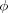A(dΣ/dΩ)A + (1−A) (dΣ/dΩ)B whereA is the volume fraction of solution A in the mixed solution (see Fig. 1). The weight-averaged SANS and SAXS intensity profiles from the homogeneous solution are found to be essentially due to incoherent scattering and thermal diffuse scattering (TDS), respectively.

### ReferencesJOURNAL OFAPPLIEDCRYSTALLOGRAPHY